MU 207 Quiz 3 Study Guide

Office hours 4:30 - 5:30 pm Tues

MacLaurin A177

Source materials:  CLASS NOTES, VIDEOS, COURSEPACK

The theory and practice of sampling/digitization: aliasing, quantization

The NYQUIST frequency is SR/2 (half the sampling rate)

If the input frequency is > 1/2 the sampling rate, the resulting frequency will be SR - input frequency.

Examples:

If the SR is 8,000 Hz, and you try to sample a sine wave with a frequency of 8,000, what will you get? -- 0 Hz (silence)
If the SR is 8,000 Hz, and you try to sample a sine wave with a frequency of 3,000, what will you get? -- 3,000 Hz (no aliasing, Nyquist is 4,000 Hz)
If the SR is 8,000 Hz, and you try to sample a sine wave with a frequency of 5,000, what will you get? -- 3,000 Hz
If the SR is 8,000 Hz, and you try to sample a sine wave with a frequency of 10,000, what will you get? -- 2,000 Hz
If the SR is 8,000 Hz, and you try to sample a sine wave with a frequency of 21,000, what will you get? -- 3,000 Hz

What is the difference between aliasing and quantization? What aspects of the sound do they determine?

Reference (not necessarily all used or referred to)• At room temperature (68° Fahrenheit), the velocity of sound in air is: 343 meters/second = 769 mph = 1,128 feet/second  = 769 miles/hour.
In water  the speed of sound is: 1,497 meters/second.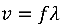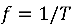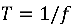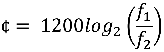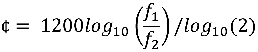(if your calculator can't do log base 2)

Rule of thumb: 6 dB increase in dynamic range per bit of quantization

1¢ is defined as 1/100th of an equal-tempered semitone